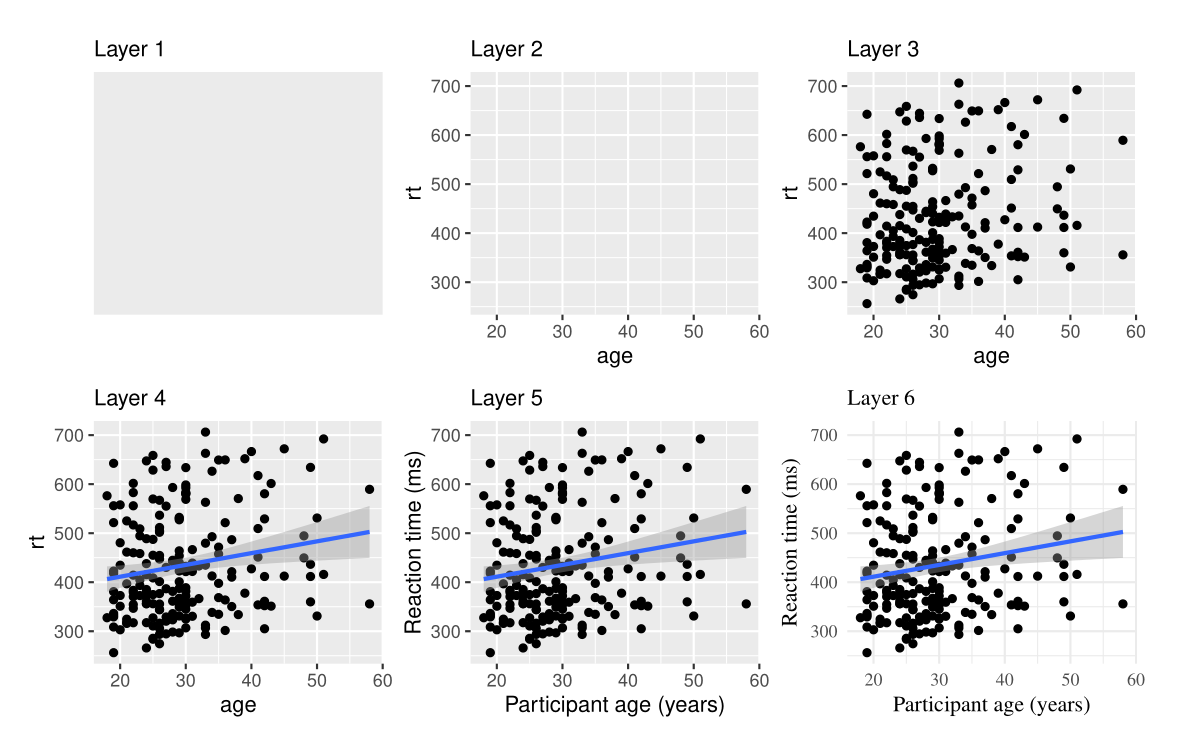# 第 14 章 数据可视化

library(tidyverse) # install.packages("tidyverse")
library(patchwork) # install.packages("patchwork")

## 14.1 为什么要可视化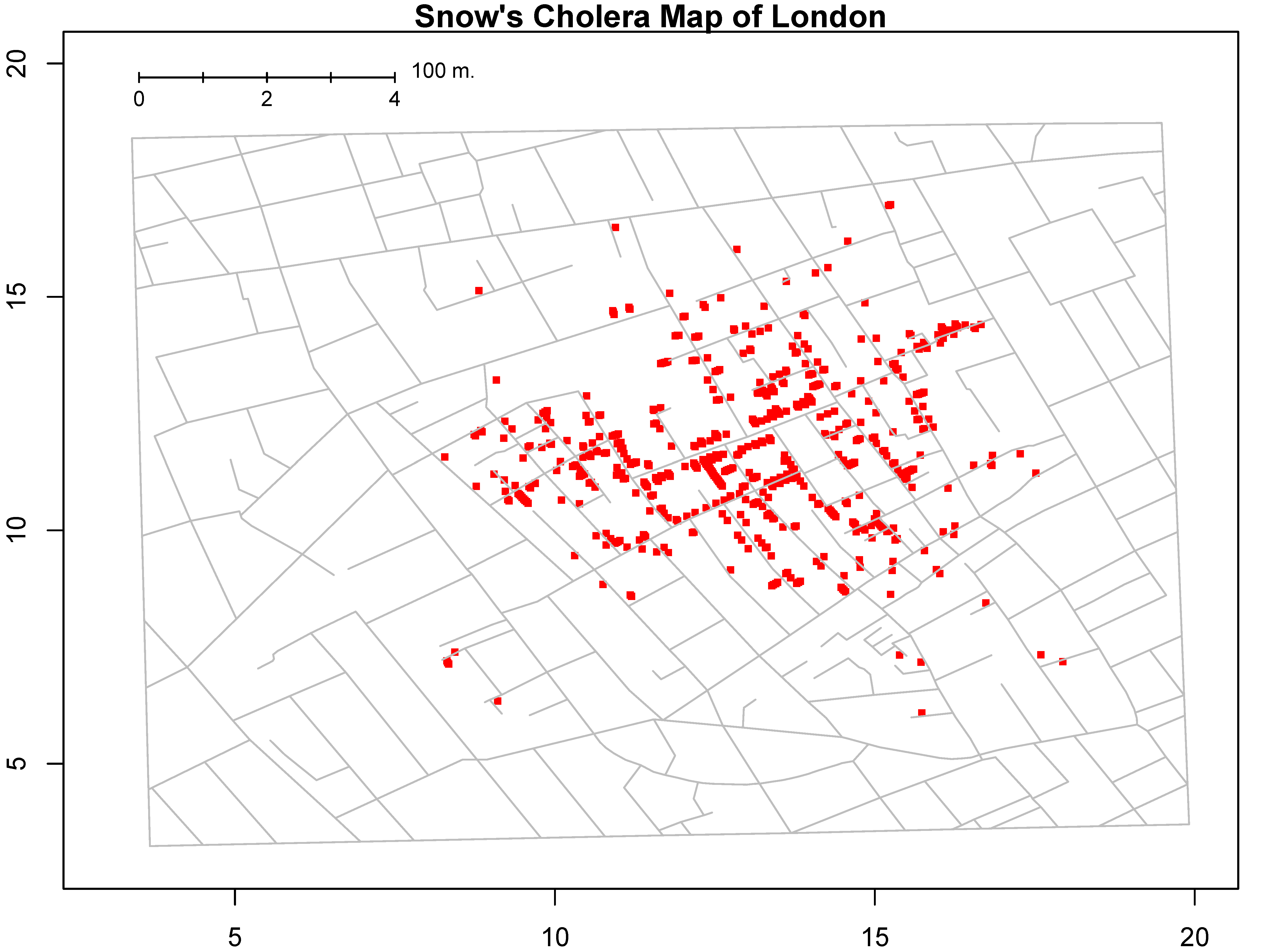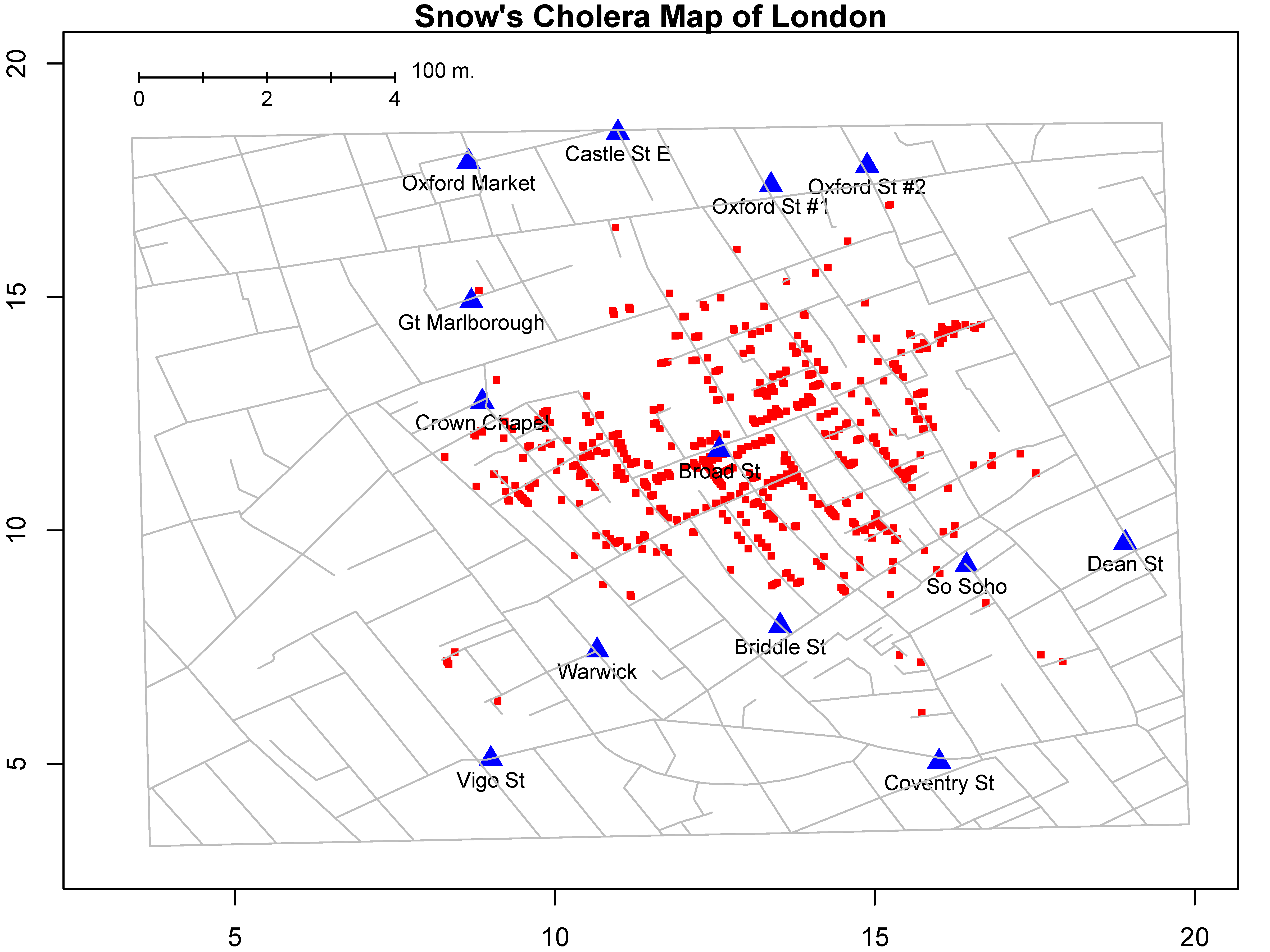## 14.2 什么是数据可视化

### 14.2.1 图形属性(视觉元素)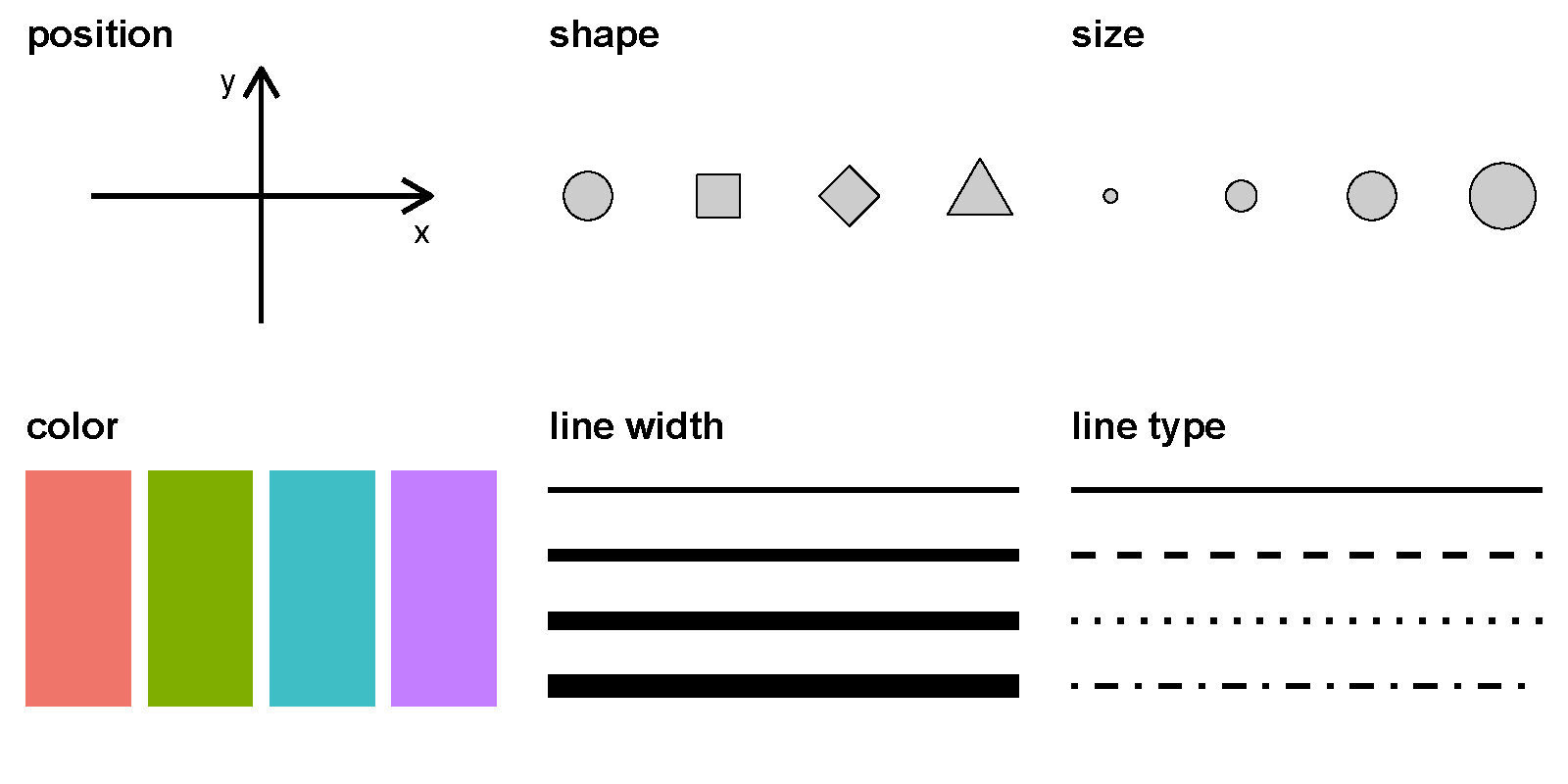geom x y size color shape linetype alpha fill group
point
line

## 14.3 宏包ggplot2

library(cranlogs)

sum(d\$count)
##  9889742

### 14.3.1 ggplot2 的图形语法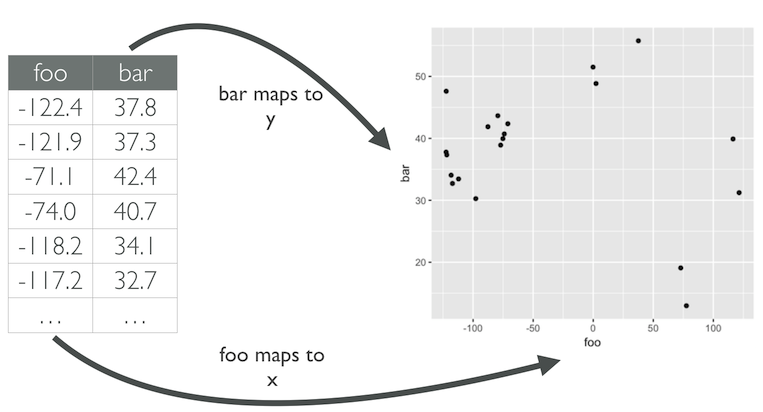### 14.3.2 怎么写代码

ggplot()函数包括9个部件：

• 数据 (data) （数据框）
• 映射 (mapping)
• 几何形状 (geom)
• 统计变换 (stats)
• 标度 (scale)
• 坐标系 (coord)
• 分面 (facet)
• 主题 (theme)
• 存储和输出 (output)

ggplot(data = <DATA>) +
<GEOM_FUNCTION>(mapping = aes(<MAPPINGS>))

library(tidyverse)
d %>% head(5)
## # A tibble: 5 × 5
##    year temp_anomaly land_anomaly ocean_anomaly
##   <dbl>        <dbl>        <dbl>         <dbl>
## 1  1880        -0.11        -0.48         -0.01
## 2  1881        -0.08        -0.4           0.01
## 3  1882        -0.1         -0.48          0
## 4  1883        -0.18        -0.66         -0.04
## 5  1884        -0.26        -0.69         -0.14
## # … with 1 more variable: carbon_emissions <dbl>
library(ggplot2)
ggplot(___) +
geom_point(
mapping = aes(x = ___, y = ___)
)

ggplot(data = d) +
geom_point(mapping = aes(x = year, y = carbon_emissions)) +
xlab("Year") +
ylab("Carbon emissions (metric tons)") +
ggtitle("Annual global carbon emissions, 1880-2014")## 14.4 映射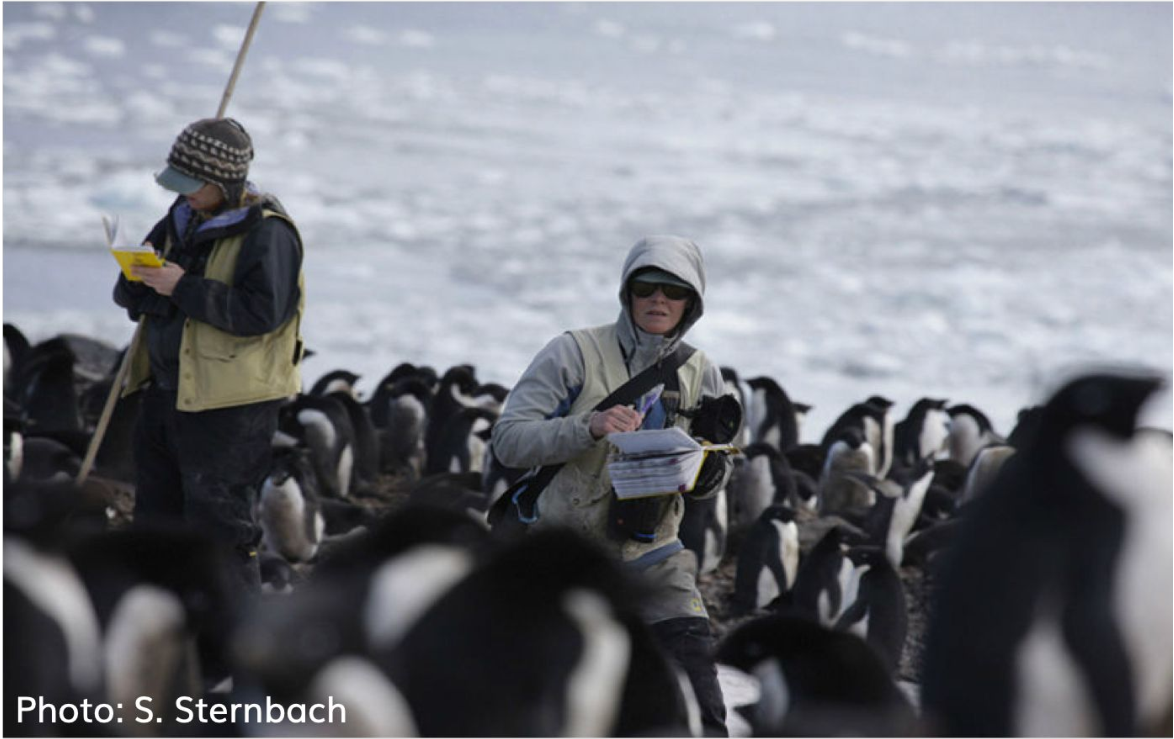library(tidyverse)
janitor::clean_names() %>%
drop_na()

penguins %>%
head()
## # A tibble: 6 × 8
##   species island    bill_length_mm bill_depth_mm
##   <chr>   <chr>              <dbl>         <dbl>
## 1 Adelie  Torgersen           39.1          18.7
## 2 Adelie  Torgersen           39.5          17.4
## 3 Adelie  Torgersen           40.3          18
## 4 Adelie  Torgersen           36.7          19.3
## 5 Adelie  Torgersen           39.3          20.6
## 6 Adelie  Torgersen           38.9          17.8
## # … with 4 more variables: flipper_length_mm <dbl>,
## #   body_mass_g <dbl>, sex <chr>, year <dbl>

### 14.4.1 变量含义

variable class description
species character 企鹅种类 (Adelie, Gentoo, Chinstrap)
island character 所在岛屿 (Biscoe, Dream, Torgersen)
bill_length_mm double 嘴峰长度 (单位毫米)
bill_depth_mm double 嘴峰深度 (单位毫米)
flipper_length_mm integer 鰭肢长度 (单位毫米)
body_mass_g integer 体重 (单位克)
sex character 性别
year integer 记录年份

penguins %>%
select(species, sex, bill_length_mm, bill_depth_mm) %>%
head(4)

### 14.4.2 嘴巴越长，嘴巴也会越厚？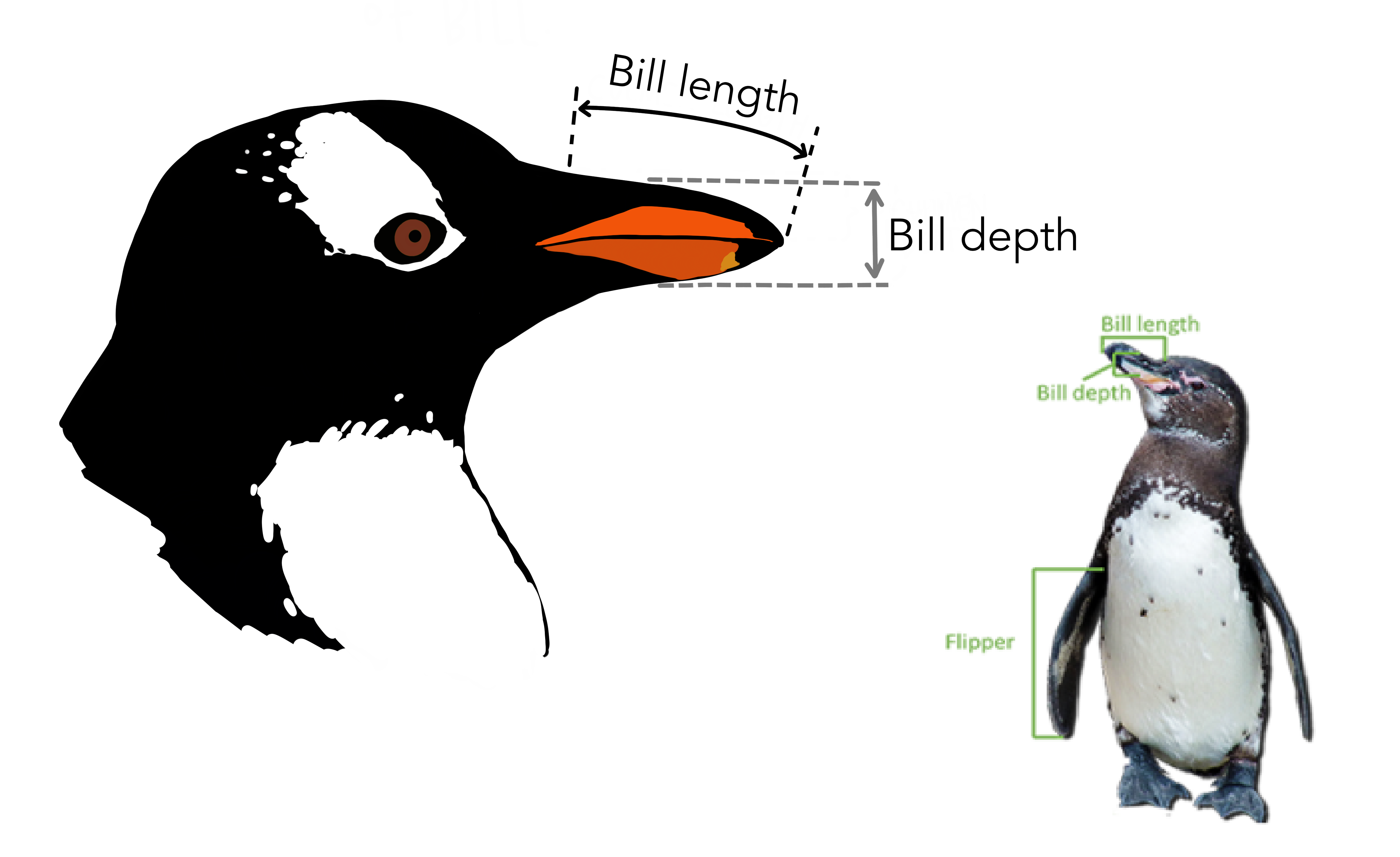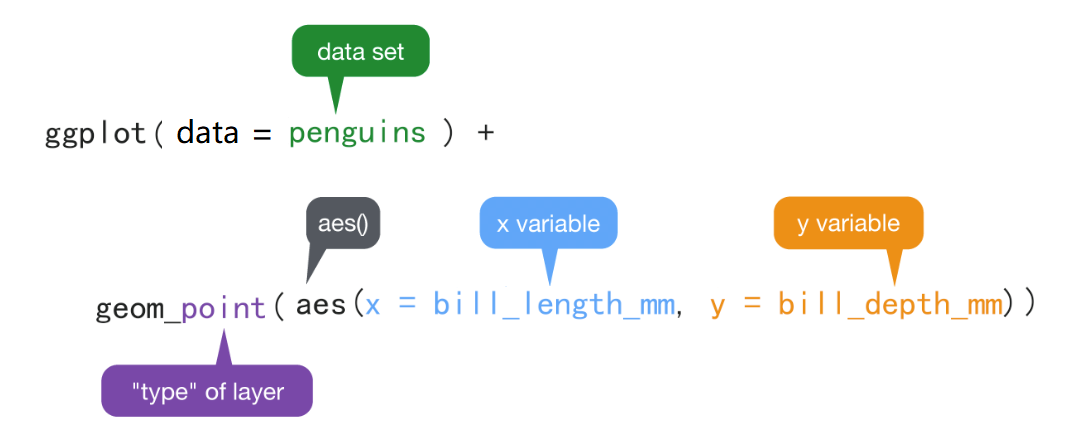• ggplot() 初始化绘图，相当于打开了一张纸，准备画画。

• ggplot(data = penguins) 表示使用penguins这个数据框来画图。

• +表示添加图层。

• geom_point()表示绘制散点图。

• aes()表示数值和视觉属性之间的映射。

aes(x = bill_length_mm, y = bill_depth_mm)，意思是变量bill_length_mm作为（映射为）x轴方向的位置，变量bill_depth_mm作为（映射为）y轴方向的位置

• aes()除了位置上映射，还可以实现色彩、形状或透明度等视觉属性的映射。ggplot(penguins) +
geom_point(aes(x = bill_length_mm, y = bill_depth_mm, color = species))ggplot(penguins) +
geom_point(aes(x = bill_length_mm, y = bill_depth_mm, size = species))
ggplot(penguins) +
geom_point(aes(x = bill_length_mm, y = bill_depth_mm, shape = species))
ggplot(penguins) +
geom_point(aes(x = bill_length_mm, y = bill_depth_mm, alpha = species))

ggplot(penguins) +
geom_point(
aes(x = bill_length_mm, y = bill_depth_mm, color = species, alpha = sex)
)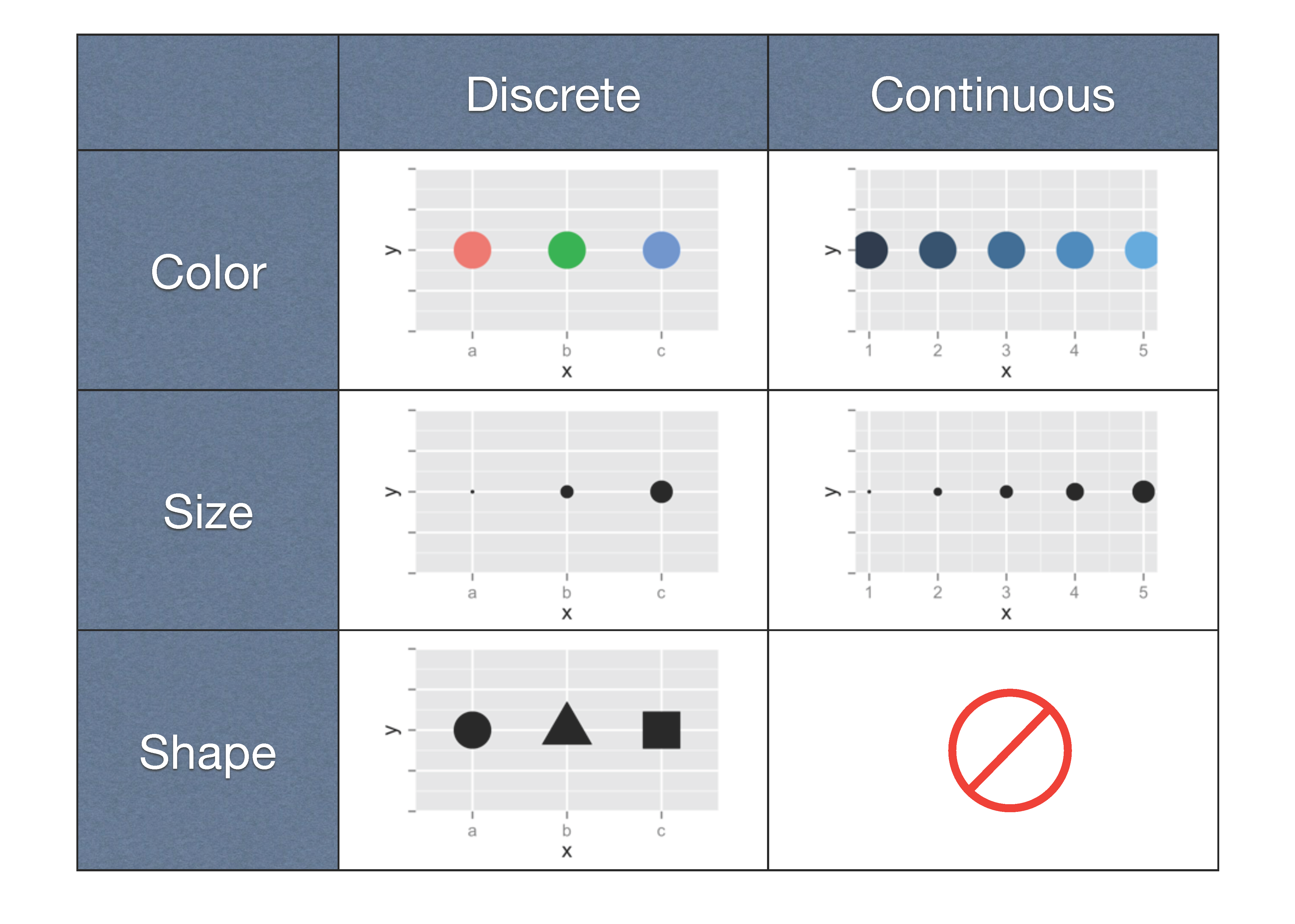## 14.5 映射 vs.设置

ggplot(penguins) +
geom_point(aes(x = bill_length_mm, y = bill_depth_mm), color = "blue")ggplot(penguins) +
geom_point(aes(x = bill_length_mm, y = bill_depth_mm), size = 5)
ggplot(penguins) +
geom_point(aes(x = bill_length_mm, y = bill_depth_mm), shape = 2)
ggplot(penguins) +
geom_point(aes(x = bill_length_mm, y = bill_depth_mm), alpha = 0.5)

### 14.5.1 提问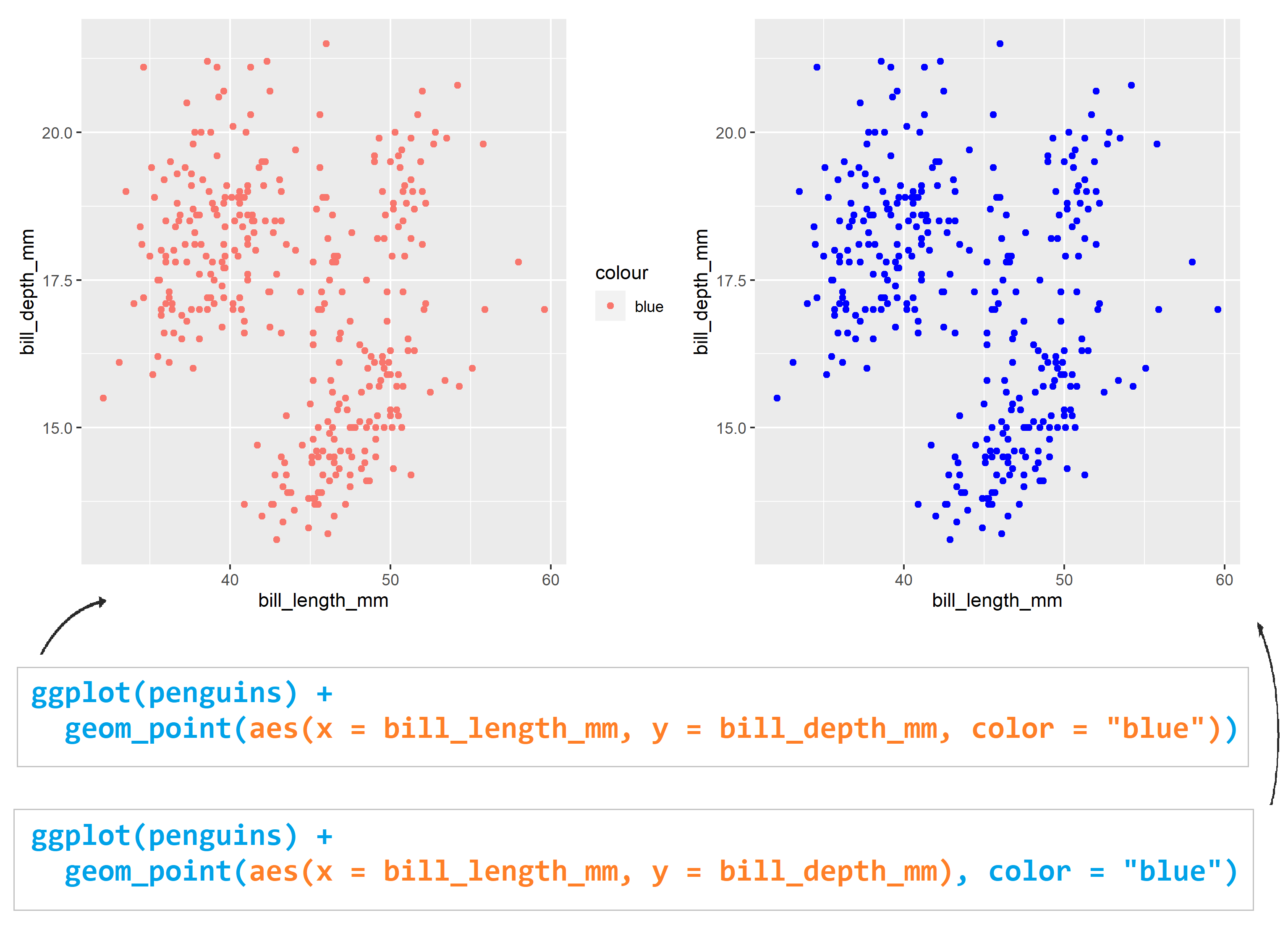## 14.6 几何形状

geom_point() 可以画散点图，也可以使用geom_smooth()绘制平滑曲线，

ggplot(penguins) +
geom_smooth(aes(x = bill_length_mm, y = bill_depth_mm))ggplot(penguins) +
geom_smooth(
aes(x = bill_length_mm, y = bill_depth_mm),
method = "lm"
)## 14.7 图层叠加

ggplot(penguins) +
geom_point(aes(x = bill_length_mm, y = bill_depth_mm)) +
geom_smooth(aes(x = bill_length_mm, y = bill_depth_mm))ggplot(penguins, aes(x = bill_length_mm, y = bill_depth_mm)) +
geom_point() +
geom_smooth()## 14.8 Global vs. Local

ggplot(penguins, aes(x = bill_length_mm, y = bill_depth_mm, color = species)) +
geom_point()ggplot(penguins) +
geom_point(aes(x = bill_length_mm, y = bill_depth_mm, color = species))• 映射关系aes(x = bill_length_mm, y = bill_depth_mm) 写在ggplot()里, 为全局声明。那么，当geom_point()画图时，发现缺少图形所需要的映射关系（点的位置、点的大小、点的颜色等等），就会从ggplot()全局变量中继承映射关系。

• 如果映射关系aes(x = bill_length_mm, y = bill_depth_mm) 写在几何形状geom_point()里, 那么此处的映射关系就为局部声明, 那么geom_point()绘图时，发现所需要的映射关系已经存在，就不会继承全局变量的映射关系。

ggplot(penguins, aes(x = bill_length_mm, y = bill_depth_mm)) +
geom_point(aes(color = species)) +
geom_smooth()

ggplot(penguins,aes(x = bill_length_mm, y = bill_depth_mm, color = species)) +
geom_point(aes(color = sex))### 14.8.1 图层从全局声明中继承

ggplot(penguins, aes(x = bill_length_mm, y = bill_depth_mm, color = species)) +
geom_point()ggplot(penguins, aes(x = bill_length_mm, y = bill_depth_mm)) +
geom_point(aes(color = species))ggplot(penguins, aes(x = bill_length_mm, y = bill_depth_mm, color = sex)) +
geom_point(aes(color = species))### 14.8.2 图层之间没有继承关系

ggplot(penguins, aes(x = bill_length_mm, y = bill_depth_mm)) +
geom_point() +
geom_smooth(method = "lm")ggplot(penguins, aes(x = bill_length_mm, y = bill_depth_mm)) +
geom_point(aes(color = species)) +
geom_smooth(method = "lm")ggplot(penguins, aes(x = bill_length_mm, y = bill_depth_mm)) +
geom_smooth(method = "lm") +
geom_point(aes(color = species))ggplot(penguins, aes(x = bill_length_mm, y = bill_depth_mm, color = species)) +
geom_point() +
geom_smooth(method = "lm")ggplot(penguins, aes(x = bill_length_mm, y = bill_depth_mm, color = species)) +
geom_point(aes(color = sex)) +
geom_smooth(method = "lm")ggplot(penguins, aes(x = bill_length_mm, y = bill_depth_mm, color = species)) +
geom_point() +
geom_smooth(method = "lm", aes(color = sex))## 14.9 保存图片

p1 <- penguins %>%
ggplot(aes(x = bill_length_mm, y = bill_depth_mm)) +
geom_smooth(method = lm) +
geom_point(aes(color = species)) +
ggtitle("This is my first plot")

ggsave(
plot = p1,
filename = "my_plot.pdf",
width = 8,
height = 6,
dpi = 300
)

penguins %>%
ggplot(aes(x = bill_length_mm, y = bill_depth_mm)) +
geom_smooth(method = lm) +
geom_point(aes(color = species)) +
ggtitle("This is my first plot")

ggsave("my_last_plot.pdf", width = 8, height = 6, dpi = 300)

## 14.10 课堂作业

• 企鹅嘴巴长度和嘴巴厚度的散点图
• 不同企鹅种类用不同的颜色
• 整体的线性拟合
• 不同种类分别线性拟合
ggplot(penguins, aes(x = ___, y = ___)) +
geom_point() +
geom_smooth() +
geom_smooth() 

## 14.11 小结